MEDIUM
DATA STRUCTURES AND ALGORITHMS

# How to Find Peak Element in an Array (With Solutions in Python, Java & JavaScript)

Written ByTom WagnerKenny Polyak

## What is the Find Peak Element Problem?

The Find Peak Element problem involves identifying the largest consecutive deltas found in a two-dimensional array of integers. To arrive at a solution, one must clearly understand the constraints of the matrix problem and apply a graph traversal algorithm to generate a linear time complexity solution.

## Examples of the Find Peak Element Interview Question

You are given a two-dimensional binary matrix where `1` represents water and `0` represents land. You can assign a height to each element in the matrix using the following rules:

• All water elements have height `0`.
• All land elements must have a non negative height.
• The largest transition between adjacent land heights can be at most 1.
• Adjacent land heights are four ways (top, bottom, left, right).

You need to assign a height to each element in the matrix such that the tallest peak is maximized, the peak being the tallest height of an element in the matrix.

Mutate the matrix in place and return the matrix with the highest peak maximized. If there are multiple solutions, return any of them.

### Example 1

Input:  Output: 

### Example 2

Input: [[0,1],[0,0]] Output: [[1,0],[2,1]]

### Example 3

Input: [[0,0,0,1,0], [0,1,0,0,0], [0,0,0,0,0]]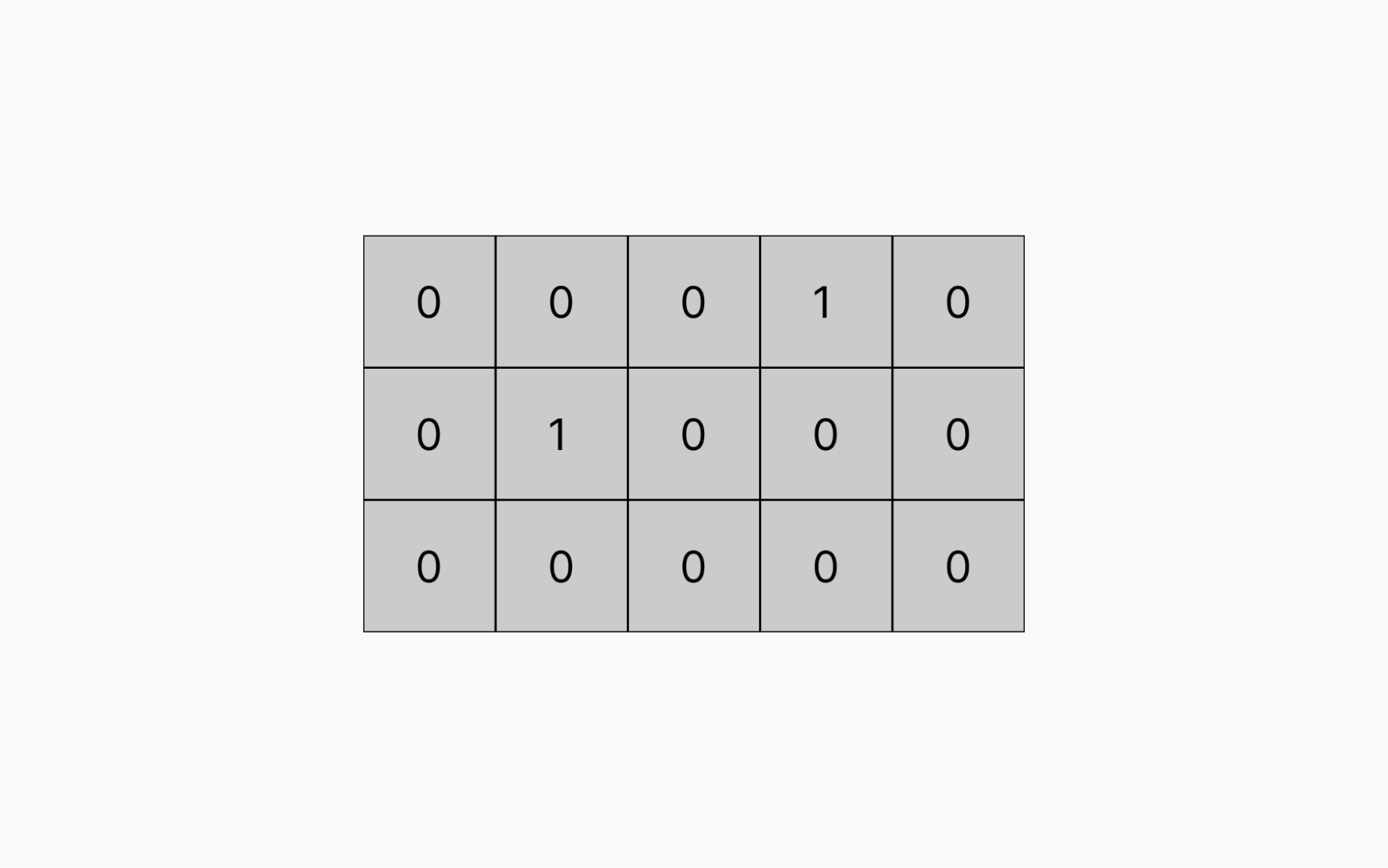Output: [[2,1,1,0,1], [1,0,1,1,2], [2,1,2,2,3]]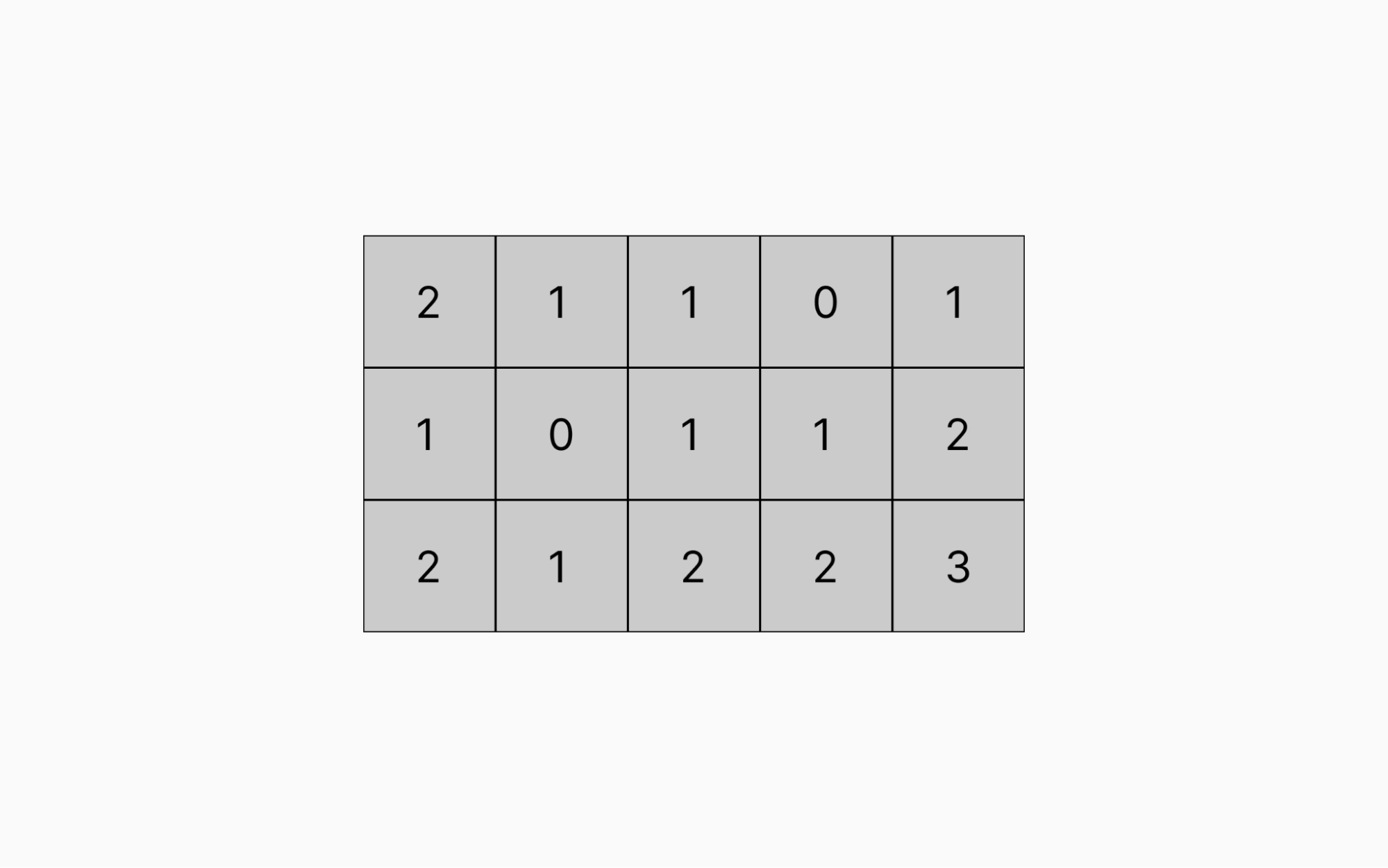Constraints

• Input matrix consists of 0s and 1s
• Input matrix is finite
• Input matrix will always contains at least one water element
• Input matrix dimensions: height = `m` and width = `n`

## How to Find the Peak Element in an Array

Below we walk through two different approaches to solving this problem: building outwards from water and storing seen locations in the input matrix.

### Approach 1: Building Outwards from Water

When tackling an algorithm problem a good place to start is to think about how you would solve the problem by hand. If given a matrix of `1`’s and `0`’s, one approach would be to start at all water locations in the matrix and “build up” the land as you go outwards from the water.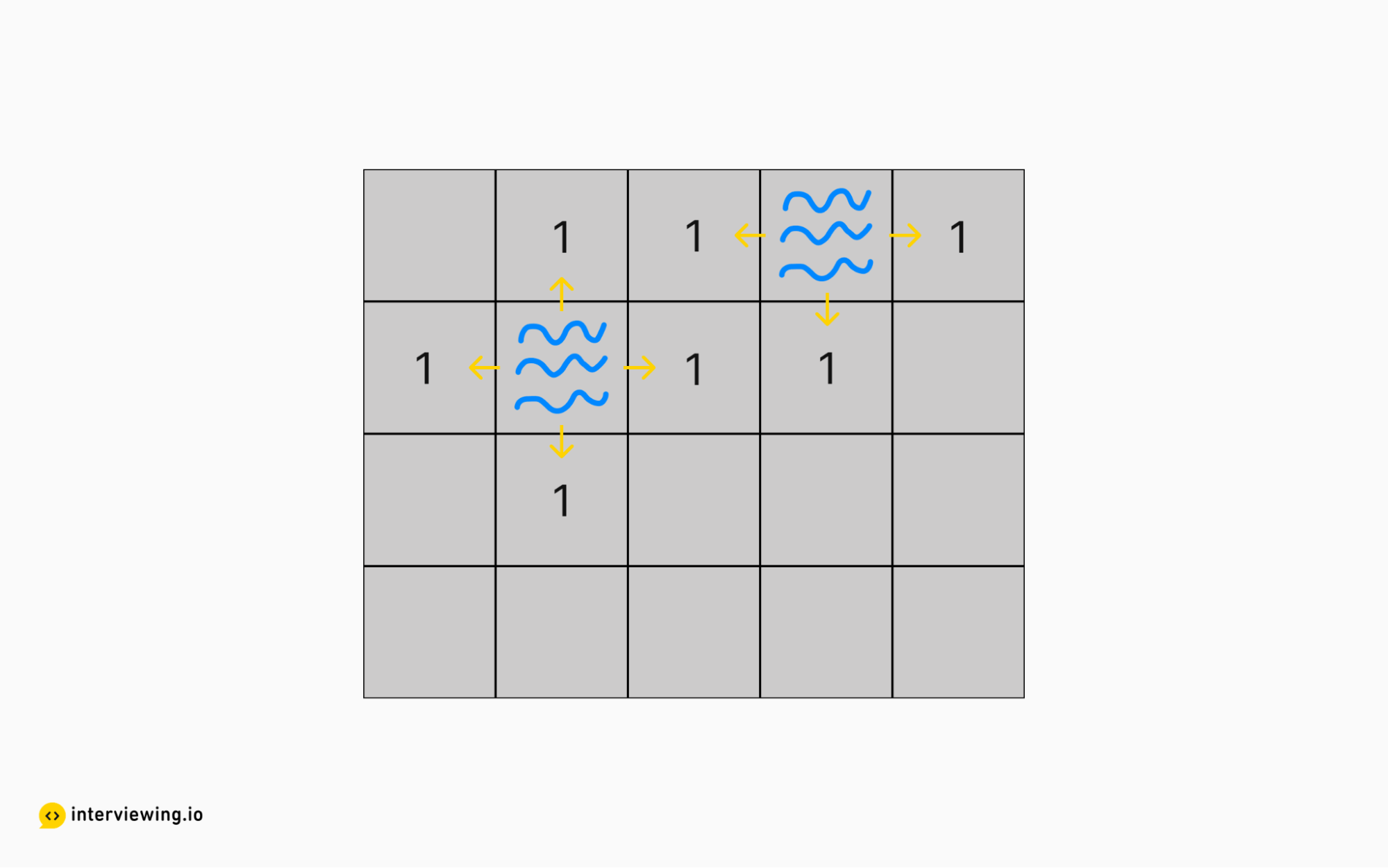We expand left, right, up and down from all water squares without going diagonally or going out of bounds.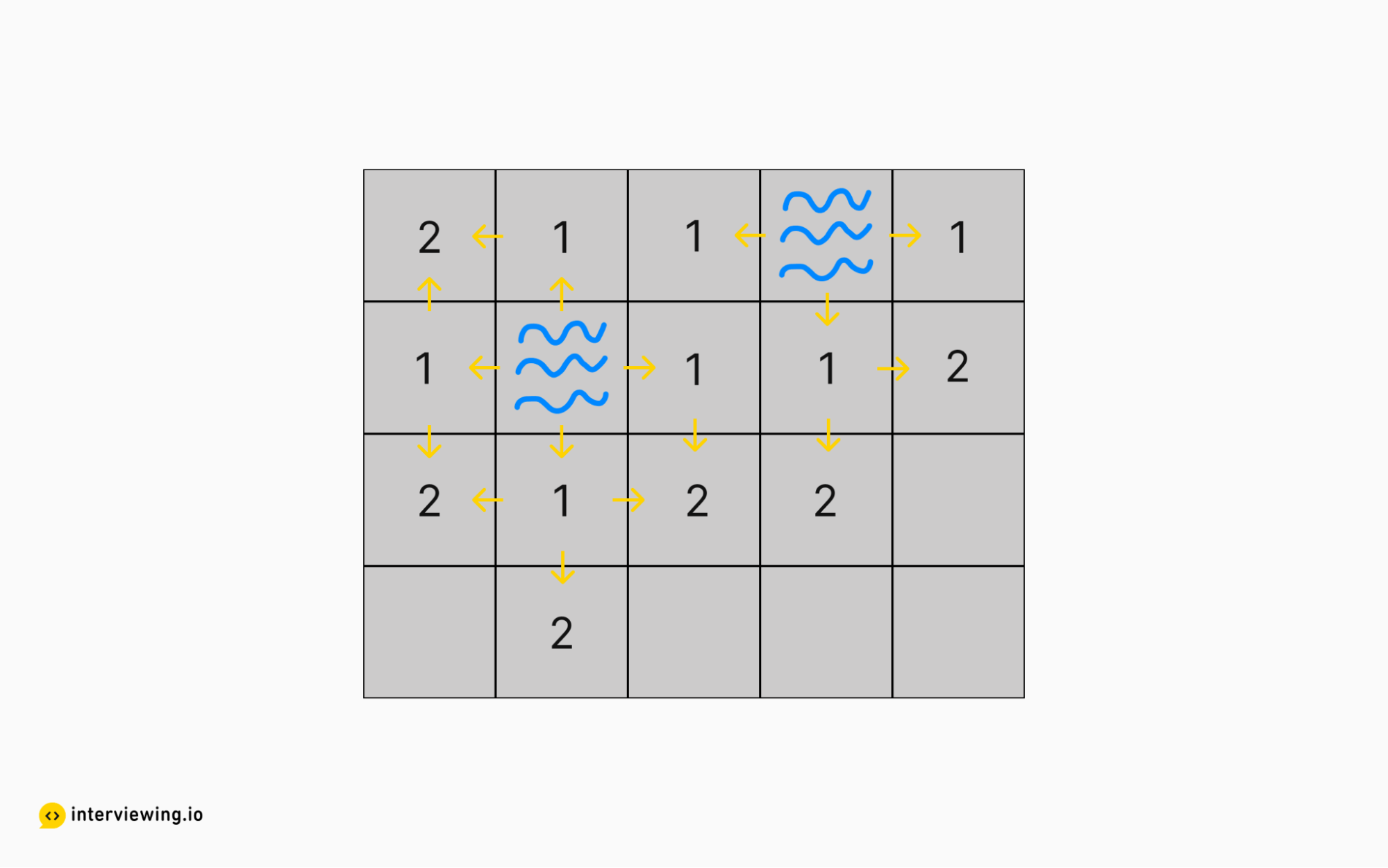We then expand outward from all the land just built. We take the value of each cell we are expanding outward from (in the above case `1`) and add `1`, as dictated by the “maximum difference of one” restraint.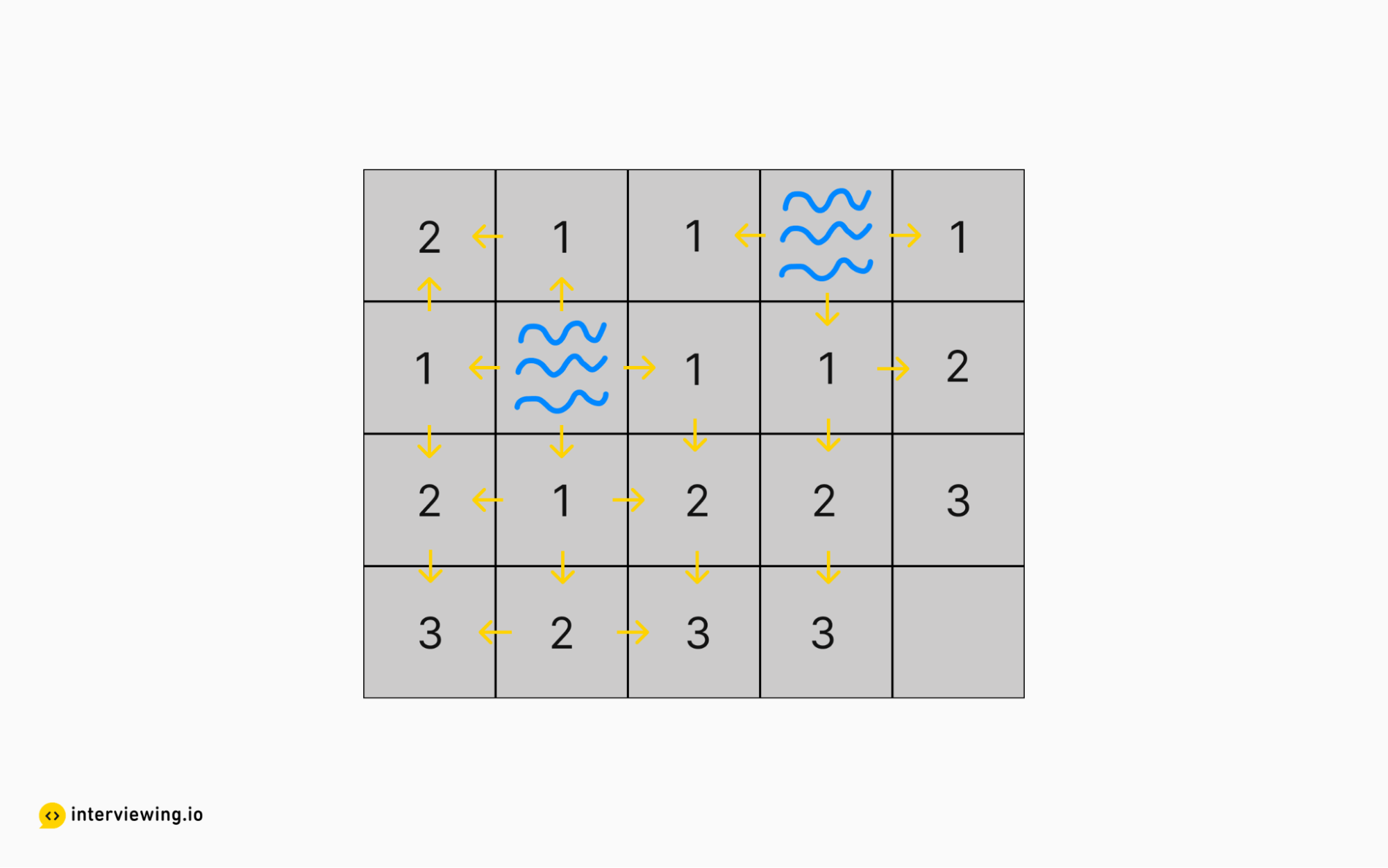We expand outwards again from all the just built `2`’s.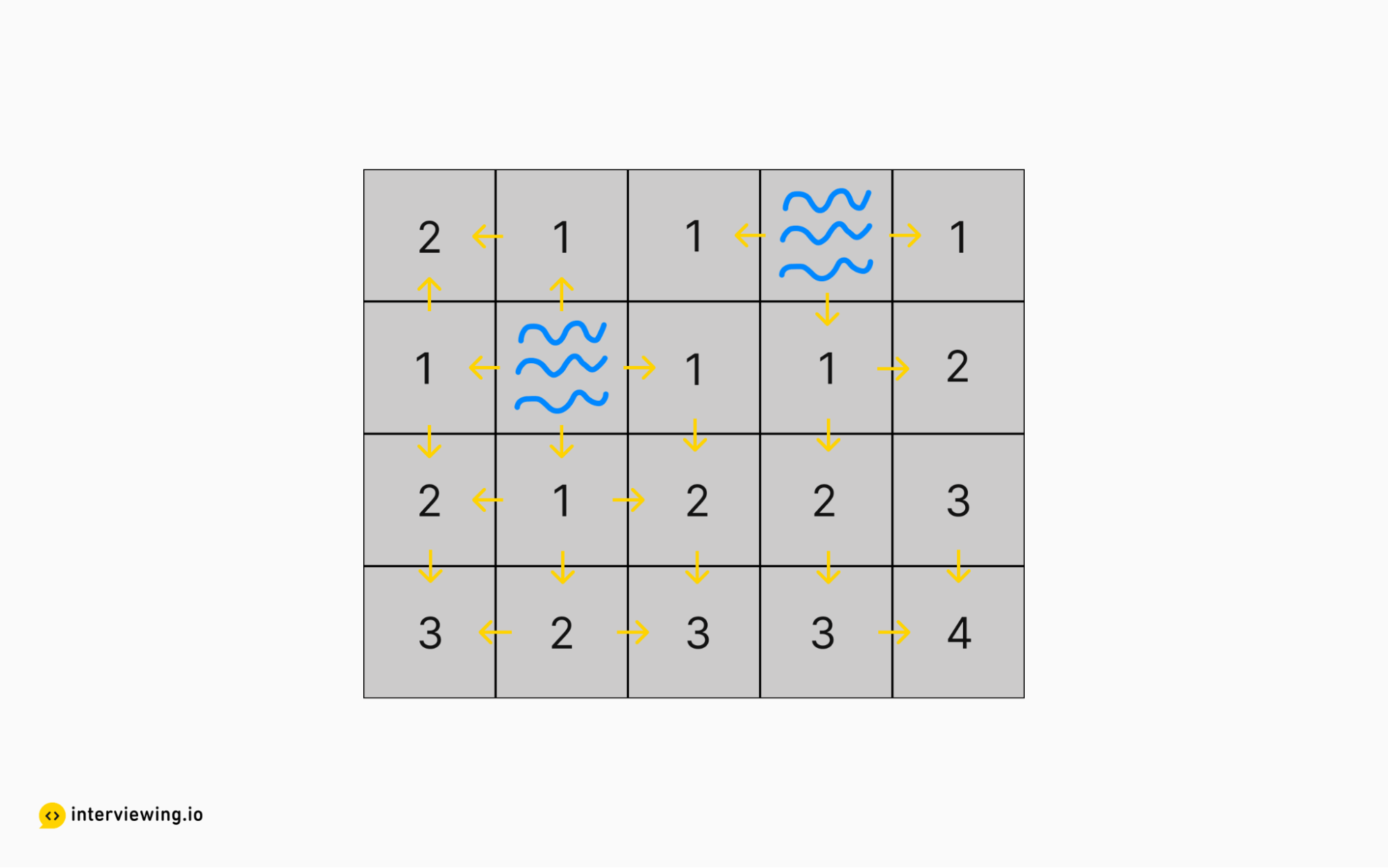And with one more expansion the matrix is filled in and height maximized.

From a code perspective, we can accomplish this approach using a queue, adding adjacent land squares that need to be processed to the queue as we move outwards from the water squares. We also keep track of locations we’ve already visited in order to avoid duplicate work.

One thing to consider when tackling this problem is whether the interviewer would rather have you mutate the matrix in place (more space efficient, but sometimes an anti-pattern to mutate inputs) vs making a copy and mutating that (which is obviously less space efficient). Acknowledging this tradeoff will likely be a positive signal to your interviewer.

#### Map of Highest Peak Python and Javascript Solution - Building Outwards from Water

``````from typing import List
import collections

class Solution:
def highestPeak(self, isWater: List[List[int]]) -> List[List[int]]:
rowMax, colMax = len(isWater), len(isWater)
seen = [[0 for _ in range(colMax)] for _ in range(rowMax)]

# standard library python data structure that acts as both a queue and a stack
queue = collections.deque()

for row in range(rowMax):
for col in range(colMax):
if isWater[row][col]:
queue.append([row, col])
seen[row][col] = 1
isWater[row][col] = 0
else:
isWater[row][col] = 1

directions = [[1, 0], [-1, 0], [0, -1], [0, 1]]
while queue:
row, col = queue.popleft()
for direction in directions:
nextRow = direction + row
nextCol = direction + col
if self.inBounds(nextRow, nextCol, rowMax, colMax) and seen[nextRow][nextCol] == 0:
isWater[nextRow][nextCol] = isWater[row][col] + 1
seen[nextRow][nextCol] = 1
queue.append([nextRow, nextCol])

return isWater

def inBounds(self, row, col, rowMax, colMax):
return not (row < 0 or col < 0 or col >= colMax or row >= rowMax)
``````

#### Time/Space Complexity

• Time complexity: `O(m*n)`, as we visit every location in the matrix once.

• Space complexity: `O(m*n)` for the matrix used to store whether we have visited a given location.

### Approach 2: Storing `Seen` Locations in the Input Matrix

This approach is just a small optimization over Approach 1. Instead of maintaining the locations which we’ve already visited in an additional 2D matrix, we can change the value in the input matrix in place to `-1` to denote locations that have already been visited.

Theoretically the matrix could consist of 99%+ water and <= 1% land, meaning 99% of the grid would end up in the queue, so the big-O space complexity is still `O(m*n)` worst case but it is much better in the average case.

#### Map of Highest Peak Python and Javascript Solutions - Storing `Seen` Locations in the Input Matrix

``````from typing import List
import collections

class Solution:
def highestPeak(self, isWater: List[List[int]]) -> List[List[int]]:
rowMax, colMax = len(isWater), len(isWater)
queue = collections.deque()

for row in range(rowMax):
for col in range(colMax):
if isWater[row][col]:
queue.append([row, col])
isWater[row][col] = 0
else:
isWater[row][col] = -1

directions = [[1, 0], [-1, 0], [0, -1], [0, 1]]
while queue:
row, col = queue.popleft()
for direction in directions:
nextRow = direction + row
nextCol = direction + col
if self.inBounds(nextRow, nextCol, rowMax, colMax) and isWater[nextRow][nextCol] == -1:
isWater[nextRow][nextCol]= isWater[row][col] + 1
queue.append([nextRow,nextCol])

return isWater

def inBounds(self, row, col, rowMax, colMax):
return not (row<0 or col<0 or col>=colMax or row>=rowMax)
``````

#### Time/Space Complexity

• Time complexity: `O(m*n)`, as we visit every location in the matrix once.
• Space complexity: `O(m*n)` because the queue length could come within one of the matrix size if ~all of the matrix were water.

## Practice the Find Peak Element in a 2D Array Problem With Our AI Interviewer

Start AI Interview